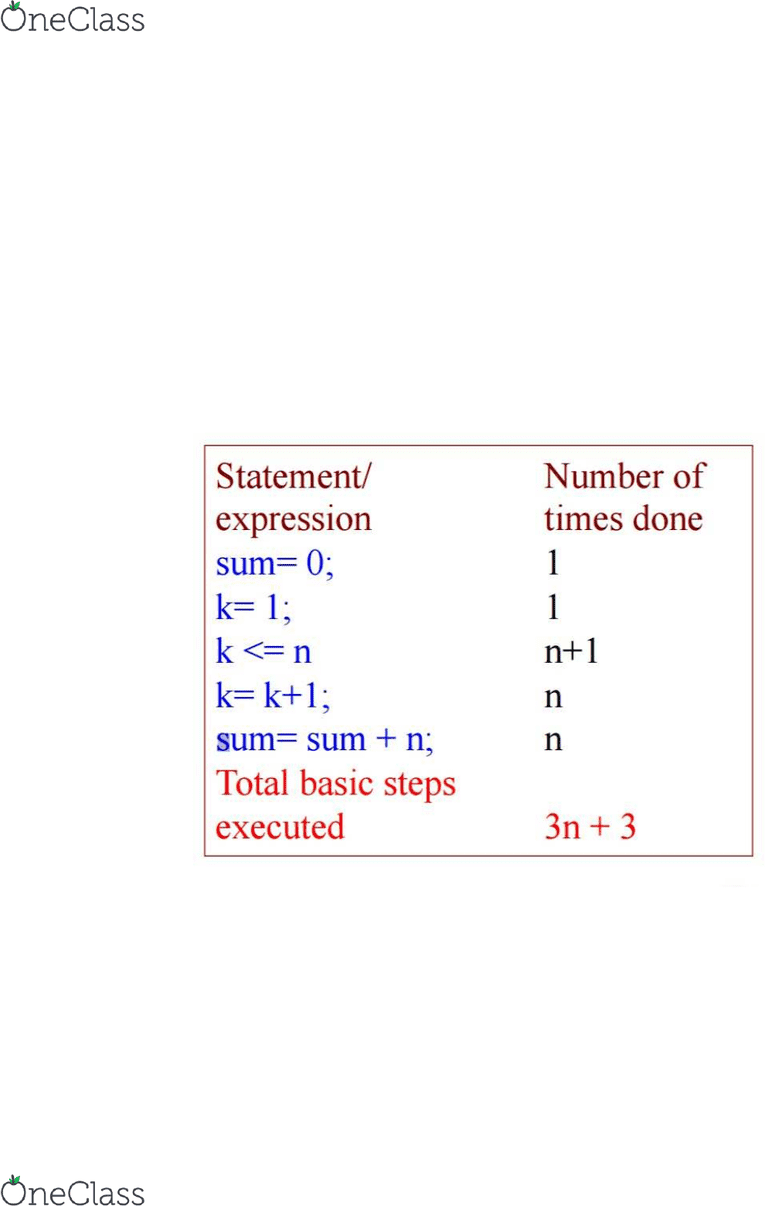Class Notes (1,100,000)
US (490,000)
Cornell (1,000)
CS (100)
CS 2110 (30)
Gries (30)
Lecture 10

# CS 2110 Lecture Notes - Lecture 10: Linear Search, Binary Search Algorithm

Department
Computer Science
Course Code
CS 2110
Professor
Gries
Lecture
10

This preview shows half of the first page. to view the full 3 pages of the document.Lecture 10 - Asymptotic complexity, searching
oWhat makes a good algorithm?
Faster
Less space
Easier to code
Easier to maintain
oBasic Step: one “constant time” operations
Constant time operation: its time doesn’t depend on the size or length of
anything. Always roughly the same. Time is bounded above by some
number
Counting basic steps in a loop
Sum = 0;
For (int k =1; k <=n; k = k+1)
Sum = sum + n
1+2+3+4…+n
Or n(n+1)/2
Linear algorithm
All operations take constant time, therefore takes time proportional
to n
oSorting:
Normal linear search
find more resources at oneclass.com
find more resources at oneclass.com